# Detect a loop in linked list using Floyd’s Cycle

July 12, 2020 7127

Given a singly Linked List, detect if it contains a loop or not.

Input: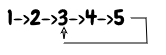Output: True

Input: 1→ 2→ 3→ NULL

Output: False

Generally, the last node of a Linked List points to a NULL pointer, which indicates the end of the Linked List. But in the Linked List containing a loop, the last node of the Linked List points to some internal node/ start node/ itself. So in such cases, we need to detect and remove the loop by assigning the next pointer of the last node to NULL. This section explains about the detection part of the loop in a Linked List.

Check below figure to visualize the Linked List containing a loop.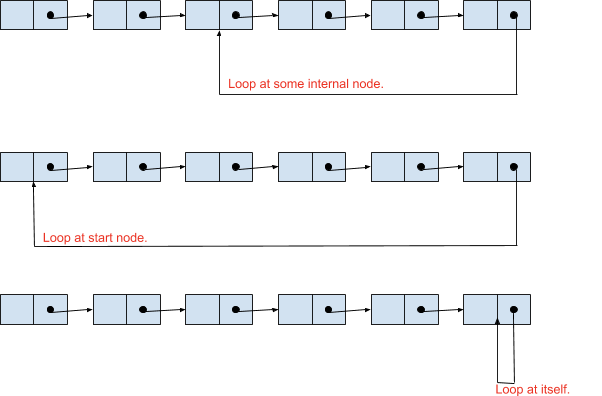## Floyd’s Cycle Finding Algorithm

Below are the steps to detect a loop in a Linked List,

1. Take two pointers ptr1 and ptr2 and initialize them to the start node.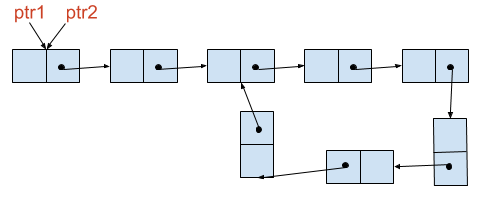2. Traverse the Linked List using both the pointers but move ptr1 one node at a time and ptr2 two nodes at a time.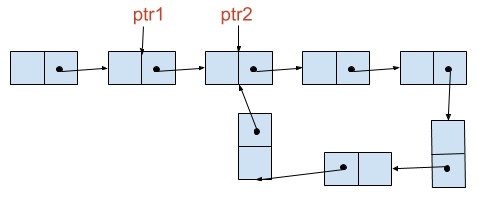3. As ptr2 is moving with double the speed, it will be ahead of ptr1. So check if ptr2 encounters NULL. If it encounters NULL, then there is no loop in the Linked List and thus stop the execution. Else ptr1 will enter the loop after some time following ptr2.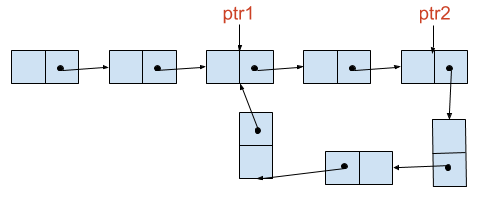4. Now, when both the pointers are in the loop and continue to move with the same speed as before, they will eventually meet at some node. And that means there is a loop in the Linked List and thus stop the execution.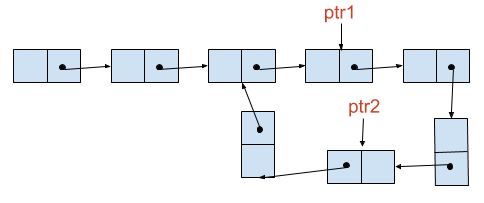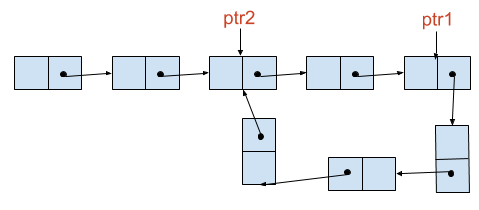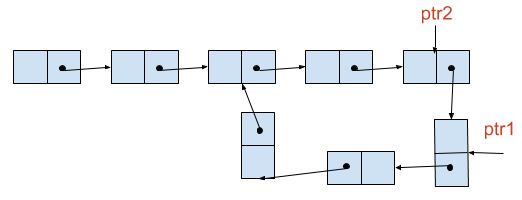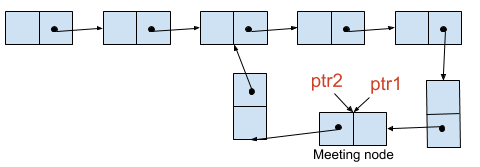The working of Floyd’s Cycle Finding Algorithm is shown below.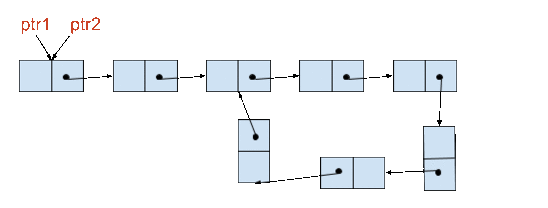The time complexity of Floyd’s Cycle Finding Algorithm is $O(n)$.

## Why pointers ptr1 and ptr2 are guaranteed to meet inside the loop?

From the below figure, let's say that after some time t, ptr1 is at x distance and ptr2 is at y distance from the starting node of the loop and the length of the loop is L.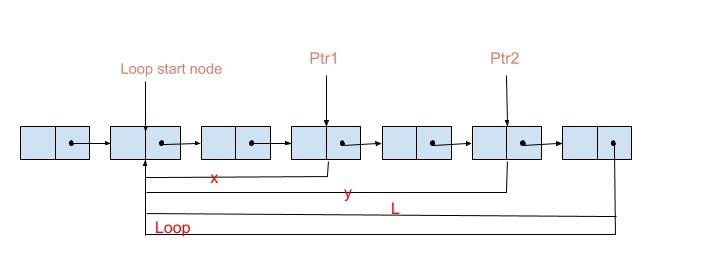Also, ptr2 is covering an extra distance of one node more as compared to ptr1 with every forward move. So every time when they move forward, the difference in their distance from the starting node of the loop, i.e. y-x increases by one node if y>x or decreases by one node if x>y. Thus eventually, y-x will become equal to L or 0, and then we can say that both the pointers are now at the same node.

Find below the implementation of Floyd’s Cycle detection algorithm,

• C/C++
• Python
• Java
#include <stdio.h>
#include <stdlib.h>
struct ListNode
{
int val;
struct ListNode *next;
};
{
// Initialize ptr1 and ptr2 to head node.
// Traverse the list until ptr2 or ptr2.next is null.
while (ptr2 != NULL && ptr2->next != NULL)
{
// Move ptr1 forward by one node.
ptr1 = ptr1->next;
// Move ptr2 forward by two nodes.
ptr2 = ptr2->next->next;
// Check if ptr1 and ptr2 meets, then there is a loop and hence return 1.
if (ptr1 == ptr2)
return 1;
}

// If ptr2 reaches to None, then there is no loop. Hence return False.
return 0;
}
int main()
{
struct ListNode *head = malloc(sizeof(struct ListNode));
struct ListNode *l1 = malloc(sizeof(struct ListNode));
l1->val = 8;
struct ListNode *l2 = malloc(sizeof(struct ListNode));
l2->val = 3;
l1->next = l2;
struct ListNode *l3 = malloc(sizeof(struct ListNode));
l3->val = 5;
l2->next = l3;
struct ListNode *l4 = malloc(sizeof(struct ListNode));
l4->val = 10;
l3->next = l4;
l4->next = l2;
// 2->8->3->5->10--
//      ^        |
//      |        |
//      |_ _ __ _|
return 0;
}

# Definition for singly-linked list.
class ListNode:
def __init__(self, x):
self.val = x
self.next = None

# Initialize ptr1 and ptr2 to head node.
while ptr2 != None and ptr2.next != None:
# Move ptr1 forward by one node.
ptr1 = ptr1.next
# Move ptr2 forward by two nodes.
ptr2 = ptr2.next.next
# Check if ptr1 and ptr2 meets, then there is a loop and hence return True.
if ptr1 == ptr2:
return True
# If ptr2 reaches to None, then there is no loop and hence return False.
return False

if __name__ == "__main__":

l1.next = l2 = ListNode(3)
l2.next = l3 = ListNode(5)
l3.next = l4 = ListNode(10)
l4.next = l2
# 2->8->3->5->10--
#       ^        |
#       |        |
#       ---------

// Definition for singly-linked list.
class ListNode {
int val;
ListNode next;

ListNode(int x) {
val = x;
next = null;
}
}

public class Solution {
public static void main(String args[]) {
ListNode l1 = new ListNode(8);
ListNode l2 = new ListNode(3);
l1.next = l2;
ListNode l3 = new ListNode(5);
l2.next = l3;
ListNode l4 = new ListNode(10);
l3.next = l4;
l4.next = l2;

// 2->8->3->5->10--
//       ^        |
//       |        |
//       ---------
}

// Initialize ptr1 and ptr2 to head node.
while (ptr2 != null && ptr2.next != null) {
// Move ptr1 forward by one node.
ptr1 = ptr1.next;
// Move ptr2 forward by two nodes.
ptr2 = ptr2.next.next;
// Check if ptr1 and ptr2 meets, then there is a loop. Hence return True.
if (ptr1 == ptr2)
return true;
}
// If ptr2 reaches to None, then there is no loop. Hence return False.
return false;
}
}


Liked the post?
0 COMMENT